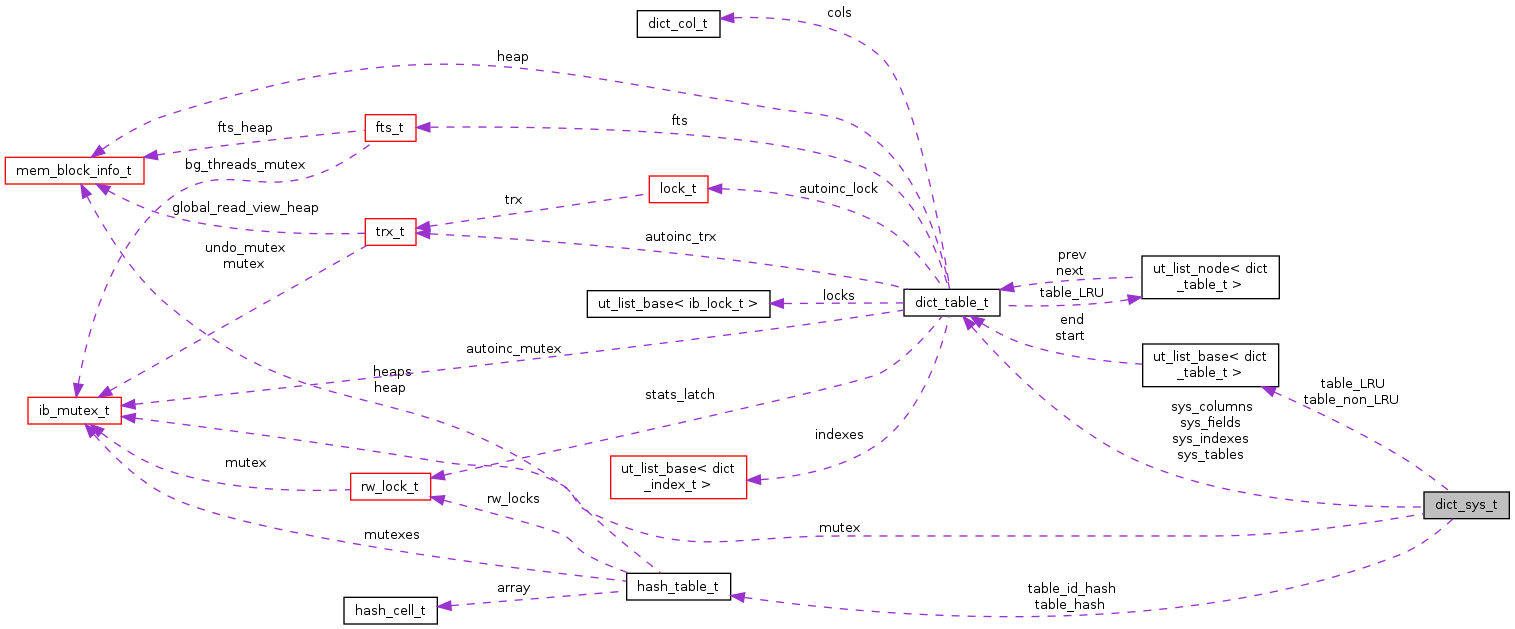InnoDB Plugin  1.0
dict_sys_t Struct Reference
Collaboration diagram for dict_sys_t:[legend]

## Data Fields

ib_mutex_t mutex
row_id_t row_id
hash_table_ttable_hash
hash_table_ttable_id_hash
ulint size
dict_table_tsys_tables
dict_table_tsys_columns
dict_table_tsys_indexes
dict_table_tsys_fields
ut_list_base< dict_table_ttable_LRU
ut_list_base< dict_table_ttable_non_LRU

## Field Documentation

 ib_mutex_t dict_sys_t::mutex

mutex protecting the data dictionary; protects also the disk-based dictionary system tables; this mutex serializes CREATE TABLE and DROP TABLE, as well as reading the dictionary data for a table from system tables

 row_id_t dict_sys_t::row_id

the next row id to assign; NOTE that at a checkpoint this must be written to the dict system header and flushed to a file; in recovery this must be derived from the log records

 ulint dict_sys_t::size

varying space in bytes occupied by the data dictionary table and index objects

 dict_table_t* dict_sys_t::sys_columns

SYS_COLUMNS table

 dict_table_t* dict_sys_t::sys_fields

SYS_FIELDS table

 dict_table_t* dict_sys_t::sys_indexes

SYS_INDEXES table

 dict_table_t* dict_sys_t::sys_tables

SYS_TABLES table

 hash_table_t* dict_sys_t::table_hash

hash table of the tables, based on name

 hash_table_t* dict_sys_t::table_id_hash

hash table of the tables, based on id

 ut_list_base< dict_table_t > dict_sys_t::table_LRU

List of tables that can be evicted from the cache

 ut_list_base< dict_table_t > dict_sys_t::table_non_LRU

List of tables that can't be evicted from the cache

The documentation for this struct was generated from the following file: